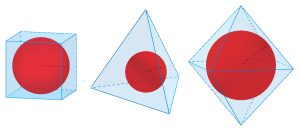(At last month’s big MathsJam conference, we asked a few people who gave particularly interesting talks if they’d like to write something for the site. A surprising number said yes. First to arrive in the submissions pile was this piece by Tom Button.)

The formula for the surface area of a sphere, $A=4\pi r^{2}$, is the derivative of the formula for the volume of a sphere: $V=\frac{4}{3}\pi r^{3}$.

This result does not hold for a cube with side length $a$ if the surface area and volume are written in terms of $a$. However, if the surface area and volume are written in terms of half the side length, $r=\frac{a}{2}$, you get the surface area $A=24 r^{2}$, which is the derivative of the volume, $V=8 r^{3}$.

If you apply a similar technique to a tetrahedron with side length $a$, you need to write the surface area and volume formulae in terms of $r=\frac{\sqrt{6}}{12}a$.  This gives $A=24\sqrt{3} r^{2}$ which is the derivative of the volume, $V=8\sqrt{3} r^{3}$.

$\frac{a}{2}$ is the inradius (radius of the insphere) of a cube with side length $a$, and $\frac{\sqrt{6}}{12}a$ is the inradius of a tetrahedron with side length $a$. This leads to a marvellous result:

The formula for the surface area is the derivative of the formula for the volume if they are both written in terms of the inradius.

The result holds for all the platonic solids. For example an octahedron with side length $a$ has inradius $r=\frac{\sqrt{6}}{6}a$. This gives surface area $A=12\sqrt{3} r^{2}$, which is the derivative of the volume, $V=4\sqrt{3} r^{3}$.I think it also works for any polyhedron whose faces are all tangent to a single sphere.

The equivalent result holds in 2D for regular polygons: the formula for the perimeter is the derivative of the formula for the area if they are both written in terms of the radius of the incircle. I think this works for non-regular polygons too, provided that all the sides are tangents to the same circle.

The original version of this was presented at the annual MathsJam conference, November 2012.  David Fontaine’s table of properties of platonic solids was very useful.

•#### Tom Button

I'm the Student Support Leader for the Further Mathematics Support Programme and Learning Technologies Specialist for MEI.

### 4 Responses to “Radii of polyhedra”

1.Andrew

I liked this talk. I’ve seen the result that the surface area is the derivative of the volume before. It isn’t coincidence; the surface of a convex shape is the infinitesimal ‘extra bit’ you keep adding to make the shape bigger.

It makes sense that it should work for any polyhedron whose faces are tangent to a sphere. The sphere is a neat way of making sure all the faces move outward in a direction normal to themselves — if they didn’t then you’d have to have cosines in there weighting things, and it would stop being so neat.

•Christian Perfect

I was going to ask if anyone could give a proof; that counts as a proof for me!

2.Adam P. Goucher

It does indeed generalise to all solids which have an insphere. Andrew’s assertions are perfectly correct, but we can prove this rigorously:

Let $V(r)$ be the volume as a function of the inradius $r$. Let $S$ and $T$ be geometrically similar polyhedra with concentric inspheres. The inradii of $S$ and $T$ are $r$ and $r+h$, respectively. Imagine extruding prisms of height $h$ on each of the faces of $S$, so that the tops of the prisms are incident with the faces of $T$. Note that the volume of the prisms is given by $Ah$, where $A$ is the surface area of the original polyhedron.

So, $V(r+h) = V(r) + Ah + \mathcal{O}(h^2)$. The $\mathcal{O}(h^2)$ term is the small excess volume near the edges of the polyhedron.

The definition of the derivative $\frac{dV}{dr}$ (evaluated at $r$) is given by the limit as $h$ tends to zero of $\frac{V(r+h)-V(r)}{h}$. This simplifies to $\frac{Ah + O(h^2)}{h} = A + \mathcal{O}(h)$. As $h$ tends to zero, this approaches $A$. Hence, the derivative $\frac{dV}{dr}$ is indeed the surface area of $S$.

•Christian Perfect